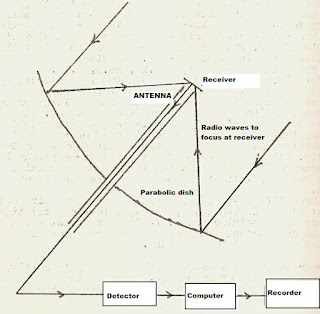Friday, November 19, 2021

The Basic Principles Of Radio Astronomy (Pt. 1- Math Included)

Examining the basic principles of radio astronomy means first recognizing we are talking about a window of observation that involves the ionosphere as opposed to the atmosphere.  In the first case we have a terrestrial layer transparent to radio waves, in the second case we have a layer transparent to light waves  - for optical observation.   The basic electromagnetic (EM)  spectrum showing the relation of visible light to radio waves is shown below:

Note here that radio waves are at much longer wavelengths.  The earliest radio wave observations were made at kilohertz (kHz) frequencies and then gradually were able to also detect sources at megahertz and beyond.   All radio astronomy - at whatever frequency- begins with knowing the basic radiation characteristics.  To this end we wish to know:

i) The direction it comes from,

ii) The emergent flux,

iii) The polarization

In respect of the flux, consider a small element of surface ds: Then to find the amount of energy which flows per unit solid angle we use the graphic below:From this we can obtain:

dE v  = I cos  q ds dw dt dv

Where:

I = f(u,f,q, x, y, z, t)

is defined as the specific intensity or brightness.  The units we are making use of here are:

[ergs / cm 2   steradian sec  cps]

Integrating the specific intensity over the solid angle:

FLUX/  F v  =    ò I v   (q,  fcos  q dw

And over the total solid angle:

F v  =   Total flux  =  ò 4p  I (q, f) dwf

F v   Units  [ergs / cm 2  sec  cps]

Note: The infinitesimal sold angle d is equivalent to:   sin q ddf

Now we examine the specific situation for a radio telescope antenna. In the most basic kind of radio telescope system we will have:Which shows a parabolic dish with the receiver at the prime focus.  Note that a parabolic dish has the property whereby radio waves reflected off it come to a focus at the same time.  This is critical given if the waves arrived at different times the telescope would be essentially useless.  The signal obtained would be incoherent which the reader might be able to deduce by the end of this post.

Note that  the antenna is essentially some sort of conducting wire or metal rod which is the 'heart' of the telescope.  Its basic function is to capture incoming electromagnetic waves and convert them into an electric current in the wire.  For maximum efficiency the dimension of the antenna wire or probe should be one fourth the size of the radio wave it is intended to intercept.  Thus for the 21 cm line of hydrogen the probe should be 5 cm in length.

There is still the matter of the parameters of the source in relation to the antenna and the solid angle formed. In the sketch below I show a radio source of area A, at a distance D from an antenna of dimension (C) and we wish to find the radio flux reaching the antenna from the source.

Comparison of solid angles, source & antenna

We have in the first instance:

dE v  = I v cos  q ds dw dE v  =

Then the flux reaching C from the radio source is:

F v  =  dE v  /  C dv dt  = I v  A W  /  C

Now, if   b is the solid angle:  A/  D 2

And  is the solid angle     C/  D 2

Then:  A/ C   =    b / W